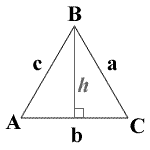## Equilateral triangle calculator

 a = P = 0 s = 0 K = 0 h = 0
» Show Steps

In geometry, Equilateral triangles have all three of their sides equal.A = angle A, B = angle B, C = angle C
a = side a, b = side b, c = side c
K = area, P = perimeter, s = semiperimeter, h = altitude

For example, A side length is a = 3:

1. To calculate P, use the following formula: 3a. When substituting a for its value, you get 3(3), which equals 9.
2. To calculate s, use the following formula: 3a/2. When substituting a and s for their values, you get 3(3)/2, which equals 4.5.
3. To calculate K, use the following formula: (1/4) * √3 * a2. When substituting a for its value, you get (1/4) * √3 * 32, which equals 3.89711.
4. To calculate h, use the following formula: (1/2) * √3 * a. When substituting a for its value, you get (1/2) * √3 * 3, which equals 2.59808.
Thinkcalculator.com provides you helpful and handy calculator resources.